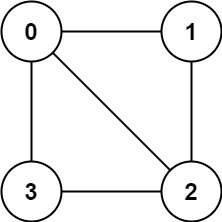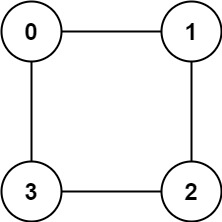# LeetCode Is Graph Bipartite Solution

There is an undirected graph with `n` nodes, where each node is numbered between `0` and `n - 1`. You are given a 2D array `graph`, where `graph[u]` is an array of nodes that node `u` is adjacent to. More formally, for each `v` in `graph[u]`, there is an undirected edge between node `u` and node `v`. The graph has the following properties:

• There are no self-edges (`graph[u]` does not contain `u`).
• There are no parallel edges (`graph[u]` does not contain duplicate values).
• If `v` is in `graph[u]`, then `u` is in `graph[v]` (the graph is undirected).
• The graph may not be connected, meaning there may be two nodes `u` and `v` such that there is no path between them.

A graph is bipartite if the nodes can be partitioned into two independent sets `A` and `B` such that every edge in the graph connects a node in set `A` and a node in set `B`.

Return `true` if and only if it is bipartite.

Example 1:Input: `graph = [[1,2,3],[0,2],[0,1,3],[0,2]]`
Output: `false`
Explanation: There is no way to partition the nodes into two independent sets such that every edge connects a node in one and a node in the other.

Example 2:Input: `graph = [[1,3],[0,2],[1,3],[0,2]]`
Output: `true`
Explanation: We can partition the nodes into two sets: `{0, 2}` and `{1, 3}`.

Constraints:

• `graph.length == n`
• `1 <= n <= 100`
• `0 <= graph[u].length < n`
• `0 <= graph[u][i] <= n - 1`
• `graph[u]` does not contain `u`.
• All the values of `graph[u]` are unique.
• If `graph[u]` contains `v`, then `graph[v]` contains `u`.

## Solution

A bipartite graph is a graph that can be paritiioned into two sets (`A` and `B`) such that every edge in the graph connects a node in `A` and a node in `B`. By traversing through the graph breadth first, we can partition the nodes into two sets, nodes in the even level and the odd level. We will specifically make sure that two nodes on the same level don't have a connection to guarantee that the graph is bipartite.

#### Implementation

Let's implement this problem by enforcing statement 3, checking whether there are links between nodes on the same level.

``````1def isBipartite(graph: List[List[int]]) -> bool:
2    visited = [False for _ in range(len(graph))]
3    def bfs(root):
4        queue = deque([root])
5        curlevel = set()
6        while queue:
7            length = len(queue)
8            newlevel = set()
9            for _ in range(length):           # for nodes in each level
10                node = queue.popleft()
11                for neighbor in graph[node]:
12                    if neighbor in curlevel:  # edge connecting nodes on the same level
13                        return False
14                    if visited[neighbor]:
15                        continue